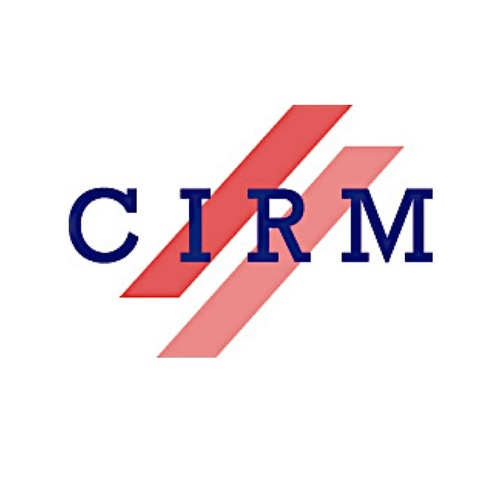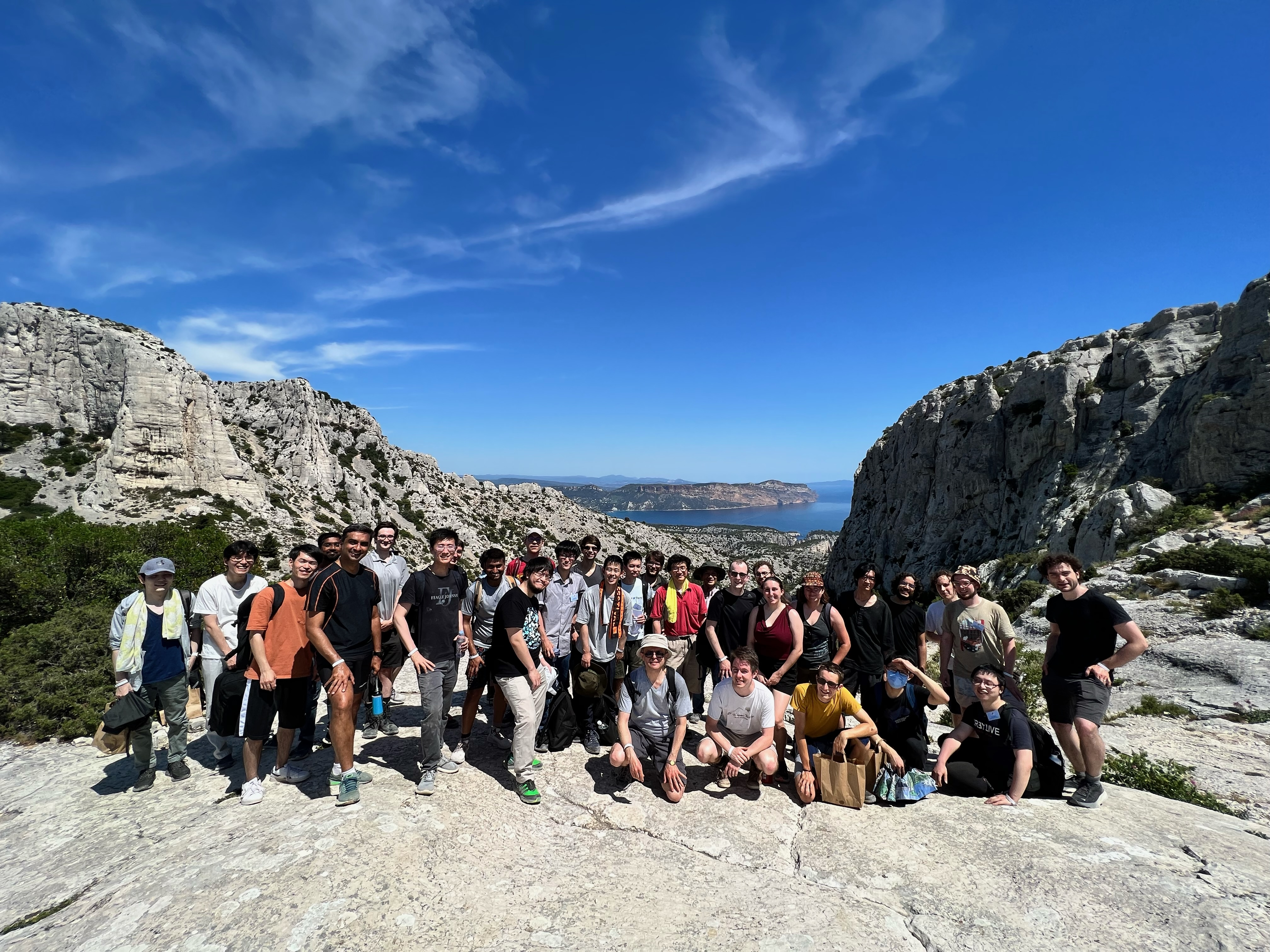Franco-Asian Summer School on Arithmetic Geometry May 30 - June 3, 2022 Centre International de Rencontres Mathématiques (CIRM), Luminy, France## Titles and abstracts

### Program and videosCourses (3 hours each)

Ahmed Abbes (CNRS & IHÉS)
Title: The p-adic Simpson correspondence: Functoriality by proper direct image and Hodge-Tate local systems
Abstract: Faltings initiated in 2005 a p-adic analogue of the (complex) Simpson correspondence whose construction has been taken up by various authors and whose properties have been developed according to several approaches. I will present in these lectures the approach I developed with Michel Gros, inspired by the Cartier transform of Ogus and Vologodsky, which is an analogue in characteristic p of Simpson correspondence. The p-adic Simpson correspondence can be considered as a categorification of the Hodge-Tate decomposition. I will present its construction for small generalized representations using a suitable period ring, establish its functoriality by proper direct image and discuss the link with Hodge-Tate local systems.
Lecture notes.

Yifeng Liu (Institute of Advanced Study in Mathematics, Zhejiang University) -- online
Title: Derivative of L-functions for unitary groups
Abstract: In this lecture series, we will focus on the recent advance on the Beilinson-Bloch conjecture for unitary Shimura varieties, more precisely, a Gross-Zagier type formula for automorphic forms on unitary groups of higher ranks. We will start from the general theory of height pairings between cycles on varieties over number fields. Then we will introduce the doubling method, a powerful tool in the theory of automorphic forms for studying L-functions for classical groups, in particular, unitary groups. Finally, we will combine the automorphic side and the arithmetic side to outline the proof of a formula relating the central derivative of L-function and the height of special cycles on Shimura varieties.
Sildes I & II, Sildes III.

Akhil Mathew (University of Chicago) -- online
Title: Some recent advances in syntomic cohomology
Abstract: Bhatt-Morrow-Scholze have defined integral refinements Z_p(i) of the syntomic cohomology of Fontaine-Messing and Kato. These objects arise as filtered Frobenius eigenspaces of absolute prismatic cohomology and should yield a theory of "p-adic étale motivic cohomology" -- for example, they are closely related to p-adic K-theory and topological cyclic homology. Moreover, they compare naturally to p-adic étale cohomology of the generic fiber. I will give an overview of some of the recent advances in the subject (due to many authors) and explain the identification (joint with Bhargav Bhatt) for regular p-torsionfree schemes.
Sildes I & II, Sildes III

Takeshi Saito (The University of Tokyo)
Title: Upper ramification groups of local fields with imperfect residue fields
Abstract: Upper ramification groups of local fields with imperfect residue fields were introduced by two of the organizers, Abbes and myself in 2000. Recently the graded quotients are shown to be F_p-vector spaces and related to Frobenius-Witt differentials. In three lectures, we outline the definition and recent developments. After short heuristic observation using rigid geometry leading to the definition, we will sketch:
1. Definition purely in the language of schemes.
2. Proof of the property that the graded quotients are F_p-vector spaces using the cotangent complexes.
3. Construction of the characteristic form relating the graded quotients to Frobenius-Witt differentials.
The third topic may be omitted depending on the time.
References:
Abbes, Saito, Ramification of local fields with imperfect residue fields, American Journal of Mathematics, 124.5 (2002), 879-920.
Saito, Wild ramification and the characteristic cycle of an l-adic sheaf, Journal de l'Institut de Mathématiques de Jussieu, (2009) 8(4), 769-829.
Saito, Graded quotients of ramification groups of local fields with imperfect residue fields, preprint https://arxiv.org/abs/2004.03768.
Lecture notes.

Lectures (1 hour each)

Ana Caraiani (Imperial College London) -- online
Title: Modularity over CM fields
Abstract: I will discuss joint work in progress with James Newton, where we prove a local-global compatibility result in the crystalline case for Galois representations attached to torsion classes occurring in the cohomology of locally symmetric spaces. I will then explain an application to the modularity of elliptic curves over imaginary quadratic fields, which also builds on recent work of Allen-Khare--Thorne.
Sildes.

Quentin Guignard (Université de Paris)
Title: Graded logarithmic geometry and valuative spaces
Abstract: We introduce a generalization of Temkin's reduction in an absolute setting. It takes the form of a category of graded log schemes, containing valuative spaces as a full subcategory, as well as more exotic objects such as the reduction mod p^n of a p-adic rigid space. We will compare the log étale and log syntomic topologies on these objects, and we will show that the ramification filtrations of Abbes-Saito, Saito and Kato-Thatte measure precisely the lack of topological invariance of the corresponding log syntomic toposes. As a byproduct, we recover and generalize results of Deligne and Hattori on the ramification of extensions of truncated discrete valuation rings.

Tongmu He (IHÉS & Université Paris-Saclay)
Title: Sen operators and Lie algebras arising from Galois representations over p-adic varieties
Abstract: Any finite-dimensional p-adic representation of the absolute Galois group of a p-adic local field with imperfect residue field is characterized by its arithmetic and geometric Sen operators defined by Sen-Brinon. We generalize their construction to the fundamental group of a p-adic affine variety with a semi-stable chart, and prove that the module of Sen operators is canonically defined, independently of the choice of the chart. When the representation comes from a Q_p-representation of a p-adic Lie group quotient of the fundamental group, we describe its Lie algebra action in terms of the Sen operators, which is a generalization of a result of Sen-Ohkubo. These Sen operators can be extended continuously to certain infinite-dimensional representations. As an application, we prove that the geometric Sen operators annihilate locally analytic vectors, generalizing a result of Pan.

Teruhisa Koshikawa (RIMS)
Title: Some cases of the Hodge standard conjecture
Abstract: The Hodge standard conjecture remains wide open. The numerical version for abelian fourfolds has been proved recently by Ancona. In this talk, I will first explain a proof of the Hodge standard conjecture for squares of K3 surfaces based on our previous work. In the remaining time, I will discuss related results and application of Ancona's idea. Joint with Kazuhiro Ito and Tetsushi Ito.

Wieslawa Niziol (CNRS & Sorbonne Université)
Title: Duality for p-adic pro-étale cohomology of analytic curves
Abstract: I will discuss duality theorems, both arithmetic and geometric, for p-adic pro-étale cohomology of rigid analytic curves. This is joint work with Pierre Colmez and Sally Gilles.

Lue Pan (Princeton University)
Title: Sen theory for locally analytic representations
Abstract: Let p be a prime number. The classical work of Sen attaches an operator (called the Sen operator) to every finite-dimensional continuous p-adic representation of the absolute Galois group of Q_p. We will present a generalization of this construction to locally analytic Galois representations (which are possibly infinite-dimensional and will be defined in the talk) and discuss several applications.

Alexander Petrov (Harvard)
Title: Automatic de Rhamness of p-adic local systems and Galois action on the pro-algebraic fundamental group
Abstract: Given a p-adic local system L on a smooth algebraic variety X over a finite extension K of Q_p, it is always possible to find a de Rham local system M on X such that the underlying local system L|_{X_{\overline{K}}} embeds into M|_{X_{\overline{K}}}. I will outline the proof that relies on the p-adic Riemann-Hilbert correspondence of Diao-Lan-Liu-Zhu.
As a consequence, the action of the Galois group G_K on the pro-algebraic completion of the étale fundamental group of X_{\overline{K}} is de Rham, in the sense that every finite-dimensional subrepresentation of the ring of regular functions on that group scheme is de Rham. This implies that every finite-dimensional subrepresentation of the ring of regular functions on the pro-algebraic completion of the geometric pi_1 of a smooth variety over a number field satisfies the assumptions of the Fontaine-Mazur conjecture. Complementing this result, I will sketch a proof of the fact that every semi-simple representation of Gal(\bar{Q}/Q) arising from geometry is a subquotient of the ring of regular functions on the pro-algebraic completion of the fundamental group of the projective line with 3 punctures.

Will Sawin (Columbia University) -- online
Title: Bounding the stalks of perverse sheaves in characteristic p via the characteristic cycle
Abstract: The sheaf-function dictionary shows that many natural functions on the F_q-points of a variety over F_q can be obtained from l-adic sheaves on that variety. To obtain upper bounds on these functions, it is necessary to obtain upper bounds on the dimension of the stalks of these sheaves. In many number theory problems, the functions we need to bound arise from perverse sheaves. David Massey proved an upper bound for the dimension of a stalk of a perverse sheaf in terms of an intersection-theoretic invariant of the characteristic cycle called a polar multiplicity. Unfortunately, he proved this only over the complex numbers, making it unusable for these problems. I will explain how to rectify this by proving an analogous statement over finite fields. This has applications to function field analogues of three problems in number theory: The Michel-Venkatesh conjecture about equidistribution of CM points, a question about large values of automorphic forms, and the number of primes in an arithmetic progression.
Sildes.

Daichi Takeuchi (Riken)
Title: On local epsilon factors of the vanishing cycles of isolated singularities
Abstract: The Hasse-Weil zeta function of a regular proper flat scheme over the integers is expected to extend meromorphically to the whole complex plane and satisfy a functional equation. The local epsilon factors of vanishing cycles are the local factors of the constant term in the functional equation. For their absolute values, Bloch proposed a conjecture, called Bloch's conductor formula, which describes them in terms of the Euler characteristics of a certain (complex of) coherent sheaf. In this talk, under the assumption that the non-smooth locus is isolated and that the residue characteristic is odd, I explain that the coherent sheaf appearing in the Bloch's conjecture is naturally endowed with a quadratic form and I would like to propose a conjecture that describes the local epsilon factors themselves in terms of the quadratic form. The conjecture holds true in the following cases: 1) for non-degenerate quadratic singularities, 2) for finite extensions of local fields, or 3) in the positive characteristic case.

Takeshi Tsuji (The University of Tokyo)
Title: Prismatic cohomology and A_inf-cohomology with coefficients
Abstract: Similarly to crystalline cohomology theory, we give a local description of a prismatic crystal and its cohomology in terms of a q-Higgs module and its q-Dolbeault complex on a bounded prismatic envelope when the base prism is defined over the prism Z_p[[q-1]]. As an application, we obtain a comparison isomorphism between A_inf-cohomology of a relative Breuil-Kisin module, studied in a joint work with M. Morrow, and prismatic cohomology of the corresponding prismatic crystal. The result also can be applied to an analogous comparison in the integral p-adic Simpson correspondence.
Lecture notes.

Daxin Xu (Chinese Academy of Sciences) -- online
Title: Parallel transport for Higgs bundles over p-adic curves
Abstract: Faltings conjectured that under the p-adic Simpson correspondence, finite dimensional p-adic representations of the geometric étale fundamental group of a smooth proper p-adic curve X are equivalent to semi-stable Higgs bundles of degree zero over X. We will talk about an equivalence between these representations and Higgs bundles whose underlying vector bundle admits potentially a strongly semi-stable reduction of degree zero. These Higgs bundles are semi-stable of degree zero and we will investigate some evidence for Faltings' conjecture.
Sildes.

Organizers

Ahmed Abbes (CNRS & IHÉS), Ana Caraiani (Imperial College London ), Ariane Mézard (Sorbonne Université),
Takeshi Saito (University of Tokyo), Takeshi Tsuji (The University of Tokyo),
Daxin Xu (Chinese Academy of Sciences), Weizhe Zheng (Chinese Academy of Sciences).

 Centre International de Rencontres Mathématiques (CIRM), 163 avenue de Luminy, Case 916, 13288 Marseille cedex 9, France For more information, send a message to abbes @ ihes.fr Registration form